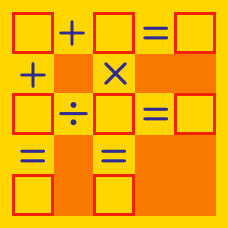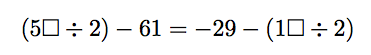Logic

# Arithmetic Puzzles - Fill in the Blanks

If $2\square + 35 = 58,$ what is $\square?$

This is an arithmetic puzzle, where $1 \square$ would represent the 2-digit number 19 if $\square = 9$. It does not represent the algebraic expression $1 \times \square$.

If $(1 \square \times 3) +8= 59,$ what number should be placed in $\square?$

This is an arithmetic puzzle, where $1 \square$ would represent the 2-digit number 19 if $\square = 9$. It does not represent the algebraic expression $1 \times \square$.

If $(5 \square \div 2) -61= -35,$ what number should be placed in $\square?$

This is an arithmetic puzzle, where $1 \square$ would represent the 2-digit number 19 if $\square = 9$. It does not represent the algebraic expression $1 \times \square$.Both boxes contain the same digit. What digit would make the equation true?

This is an arithmetic puzzle, where $1 \square$ would represent the 2-digit number 19 if $\square = 9$. It does not represent the algebraic expression $1 \times \square$.

If $2 \square \times 4 = 88,$ what number should be placed in $\square?$

This is an arithmetic puzzle, where $1 \square$ would represent the 2-digit number 19 if $\square = 9$. It does not represent the algebraic expression $1 \times \square$.

×